## 26) Bonds

Bonds of 10: Bonds Building a Wall

### Mathematics

Develop the concept of:

• The whole of 10 being equal to two parts joined together.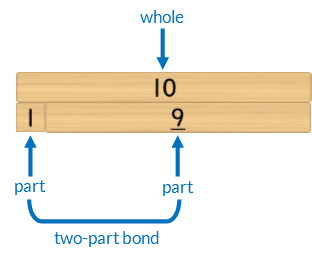The two parts bond (join) together to become equal to the length of the whole.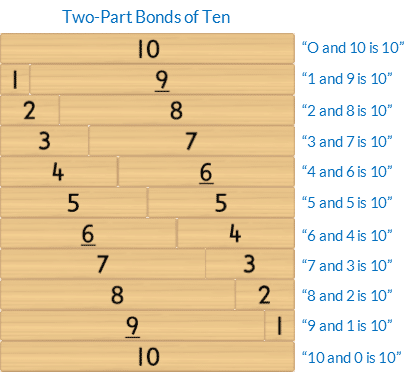• The Commutative Property of Addition: swapping the order of the parts does not alter the size of the whole. For example, changing the order of the parts of 3 and 2  to 2 and 3 does not alter the size of the whole.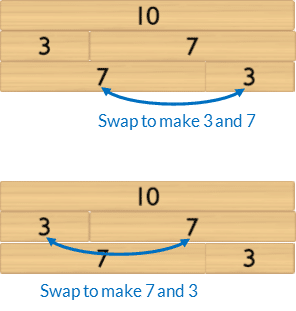• Mathematics as the science of pattern.

### Language

• “(Part) and (part) is (whole)”. eg “4 and 6 is 10”
• addition as “and” when joining parts
• equals as “is”, “is equal to”
• bond
• too long, too big, too short, too small
• commutative property
• row (horizontal)

### Core Activity Support MaterialsClick to open in a new tab.## Differentiation

### A little easier

##### Counting order

Scaffold finding the Bonds of 10 in counting order.

• Place the 10 block horizontally in front of the student.
• Then place the 1 block underneath the 10. Ask the student, “Which number joins with 1 to make it the same as 10?” Model saying the bond, whilst touching the related blocks, “Yes, 1 and 9 is 10”
• Repeat this process with each block in counting order.

### A little harder

##### Fluency packing away

Develop fluency with bonds of 10 when packing away. Cover the template with a piece of white paper. Students pick up one block randomly then find its bond of ten.

##### Three-part bonds of five

Instruct students to build one row of ten with three blocks (parts).

• Select one row.
• Work mathematically by moving the blocks in a systematic order to list all the addition combinations for that bond.

For example, 1+2+7 is equal to 1+7+2 and 2+1+7 and 2+7+1 and 7+1+2 and 7+2+1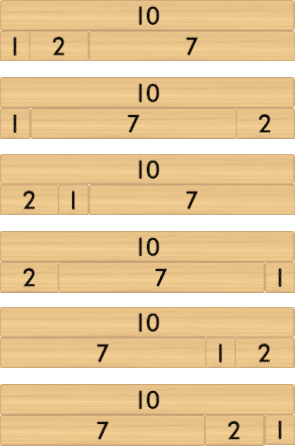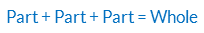The associative property of addition: three or more parts can be added in any order without changing the size of the whole.

### Progression

In the next activity students develop fluency with bonds of 10. Go to

##### Activity 27

Bonds of 10: Fluency, Filling a Wall